﻿ 交叉口电动自行车群体过街特性 Crossing Characteristics of Electric Bicycle Groups at Intersections

Open Journal of Transportation Technologies
Vol.07 No.03(2018), Article ID:25059,7 pages
10.12677/OJTT.2018.73023

Crossing Characteristics of Electric Bicycle Groups at Intersections

Qiong Peng1, Tao Wang1, Wenyong Li1,2, Bizhong Wei1

1School of Architecture and Transportation, Guilin University of Electronic Technology, Guilin Guangxi

2Guangxi Intelligent Transportation System key Laboratory, Guilin Guangxi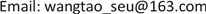Received: May 3rd, 2018; accepted: May 17th, 2018; published: May 24th, 2018ABSTRACT

On the basis of actual measurement data of electric bike group crossing behavior, using mathematical statistics methods, the characteristics of speed, density and number within the group of electric bicycle crossing groups at signalized intersections were first studied; secondly, the correlation between speed and density of electric bicycles was discussed. Sexuality was analyzed and a speed-density relationship model was established. The results show that there are 4 - 8 individuals in the number of electric bicycles crossing the street. The distribution of density is mainly in saddle type, mainly distributed in 0.22 - 0.38 vehicles/m2 (88%). The group of electric cyclists obeys the normal distribution at the intersection speed. The main distribution is in the range of 11 - 13 km/h.

Keywords:Intersection, Electric Bicycles, Communities across the Street

1桂林电子科技大学，建筑与交通工程学院，广西 桂林

2广西智能交通系统重点实验室，广西 桂林1. 引言

2. 数据采集与提取

${x}_{r}$${y}_{r}$ 为道路坐标， ${x}_{s}$${y}_{s}$ 为屏幕坐标， ${c}_{1}\sim {c}_{8}$ 为转换系数，满足：

${x}_{r}=\frac{{C}_{1}+{C}_{2}{x}_{s}+{C}_{3}{y}_{s}}{{C}_{4}{x}_{s}+{C}_{5}{y}_{s}+1}$ (1)

${y}_{r}=\frac{{C}_{6}+{C}_{7}{x}_{s}+{C}_{8}{y}_{s}}{{C}_{4}{x}_{s}+{C}_{5}{y}_{s}+1}$ (2)

$\left[\begin{array}{c}{x}_{r,1}\\ {x}_{r,2}\\ {x}_{r,3}\\ {x}_{r,4}\\ {y}_{r,1}\\ {y}_{r,2}\\ {y}_{r,3}\\ {y}_{r,4}\end{array}\right]=\left[\begin{array}{cccccccc}1& {x}_{s,1}& {y}_{s,1}& -{x}_{s,1}{x}_{r,1}& -{y}_{s,1}{x}_{r,1}& 0& 0& 0\\ 1& {x}_{s,2}& {y}_{s,2}& -{x}_{s,2}{x}_{r,2}& -{y}_{s,2}{x}_{r,2}& 0& 0& 0\\ 1& {x}_{s,3}& {y}_{s,3}& -{x}_{s,3}{x}_{r,3}& -{y}_{s,3}{x}_{r,3}& 0& 0& 0\\ 1& {x}_{s,4}& {y}_{s,4}& -{x}_{s,4}{x}_{r,4}& -{y}_{s,4}{x}_{r,4}& 0& 0& 0\\ 0& 0& 0& -{x}_{s,1}{y}_{r,1}& -{y}_{s,1}{y}_{r,1}& 1& {x}_{s,1}& {y}_{s,1}\\ 0& 0& 0& -{x}_{s,2}{y}_{r,2}& -{y}_{s,2}{y}_{r,2}& 1& {x}_{s,2}& {y}_{s,2}\\ 0& 0& 0& -{x}_{s,3}{y}_{r,3}& -{y}_{s,3}{y}_{r,3}& 1& {x}_{s,3}& {y}_{s,3}\\ 0& 0& 0& -{x}_{s,4}{y}_{r,4}& -{y}_{s,4}{y}_{r,4}& 1& {x}_{s,4}& {y}_{s,4}\end{array}\right]\left[\begin{array}{c}{C}_{1}\\ {C}_{2}\\ {C}_{3}\\ {C}_{4}\\ {C}_{5}\\ {C}_{6}\\ {C}_{7}\\ {C}_{8}\end{array}\right]$ (3)

$k=\frac{{\sum }_{i=1}^{30}{k}_{i}}{30}\cdot \frac{1}{L\cdot W}$ (4)

3. 信号交叉口电动自行车群体内个体数、过街密度、速度特性

3.1. 电动自行车群内个体数分布特性

3.2. 交叉口电动自行车过街群体密度分布特性

1) 信号交叉口处的停车区域因素。由于受红灯时间的限制，随机到达时在停车线上以群的形式聚集。绿灯开启后，受启动时间以及延误的影响时间各不相同，在绿灯初期就已经出现车群面积膨胀现象，在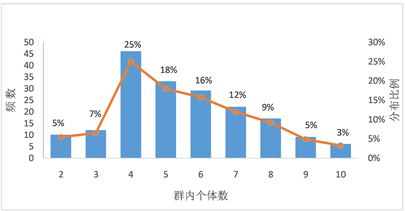Figure 1. Characteristics of individual number distribution in a group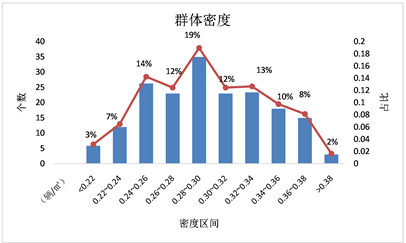Figure 2. Density distribution characteristics

2) 骑行者自身的主观因素。速度不一使得群体出现“两级分化”，这是每一个绿灯时间内过街的电动自行车群体几乎都会出现的情况。在绿灯时间内，由于电动自行车的抵达具有随机性和集群性，绿灯畅行时受到的延误较小，骑行者的抢行心理等使得过街速度较快，结群面积相对较大，所以这就造成了密度较小，这种现象微观上就产生密度较小的情况。

3) 群内的个体数量因素。在群体的划分中。按照群的定义划分的群体大小不一致，直观上而言，群体小速度较快，其骑行所需的电动自行车的行驶侧向净宽就大，占地面积大。与之对应的，单个群体个数多，其个体间的速度分布离散小，集群占地面积相对较小。同时，群体的个体间也有差异，所以就出现了密度较大的情况。

3.3. 交叉口电动自行车群过街速度分布特性

4. 电动自行车群体速度与密度关系模型

$y={v}_{0}{\text{e}}^{{k}_{0}x}$

$y=35.786{\text{e}}^{-2.563x}$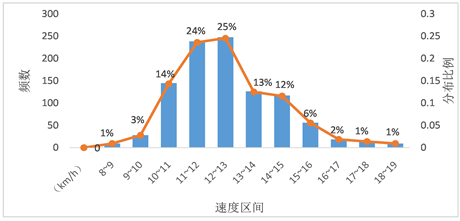Figure 3. Velocity distribution diagram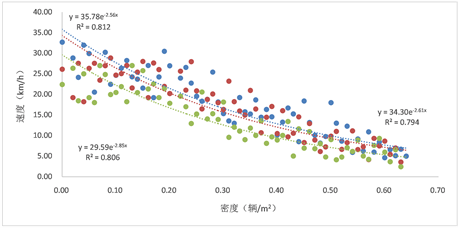Figure 4. Velocity-density scatter plotTable 1. Coefficient table of velocity and density model

5. 总结

1) 利用视频检测实测数据对信号交叉口电动自行车群体过街特性进行了研究，分别对信号交叉口电动自行车群体密度、速度特性进行分析，发现交叉口过街群体速度与密度之间呈显著负相关；

2) 密度的分布宏观上表现为马鞍型，主要分布在0.22~0.38辆/m2 (占比88%)；

3) 速度分布相对集中，绿灯亮起后电动自行车群通过交叉口的速度具有一定的离散性，经验证服从正态分布，离散程度较小，分布范围小；

4) 对密度与速度的分析发现，他们之间呈高度显著负相关；并对密度和速度特性进行量化研究，提出电动自行车群体的密度与速度关系模型。

Crossing Characteristics of Electric Bicycle Groups at Intersections[J]. 交通技术, 2018, 07(03): 182-188. https://doi.org/10.12677/OJTT.2018.73023

1. 1. Lam, W.H.K., Lee, J.Y.S. and Cheung, C.Y. (2002) A Study of the Bi-Directional Pedestrian Flow Characteristics at Hong Kong Signalized Crosswalk Facilities. Transportation, 29, 3-6.

2. 2. Bennett, S., Felton, A. and Akcelik, R. (2010) Pede-strian Movement Characteristics at Signalized Intersections. 23rd Conference of Australian Institutes of Transport Research (CAITR), Melbourne, 10-12 December 2001, 4-7.

3. 3. 周致纳, 史忠科, 李迎峰. 行人群体闯红灯行为决策模型[J]. 系统工程理论与实践, 2009, 29(11): 177-182.

4. 4. 赵莹莹, 曲昭伟, 江晟, 等. 基于视频的行人过街速度特性[J]. 吉林大学学报(工学版), 2013, 43(2): 298-303.

5. 5. 李新波. 基于博弈论的路段行人过街决策研究[D]: [硕士学位论文]. 长春: 吉林大学, 2016.

6. 6. 陈明涛. 路段行人过街特性及其控制方法研究[D]: [硕士学位论文]. 长春: 吉林大学, 2016.

7. 7. 董艳涛. 城市道路电动自行车交通特性研究[D]: [硕士学位论文]. 镇江: 江苏大学, 2016.

8. 8. 韦凌翔, 王永岗, 李文文, 等. 城市道路交叉口电动自行车左转安全特性分析[J]. 盐城工学院学报(自然科学版), 2017, 30(2): 11-15.

9. 9. 于泉, 史丽平, 李宁. 信号交叉口自行车群通行阶段划分[J]. 交通运输系统工程与信息, 2011, 11(4): 135-139.

10. 10. 周晨静, 荣建, 冯星宇. 信号交叉口引道自行车群释放速度统计模型[J]. 道路交通与安全, 2015, 15(2): 38-41.

11. 11. Brambilla, M., Ceri, S., Facca, F.M., et al. (2007) Model Driven Design and Development of Semantic Web Service Application. ACM Transactions on Internet Technology (TOIT), 77, 225-248.